# How to Find Max And Min Value with Single or Multiple Criteria in Excel

This post will guide you how to find the maximum value in a range of cells based on single or multiple criteria in Excel. How do I calculate the Minimum value based on criteria with MIN Function in Excel.

## Find Max Value based on Single Criteria

Assuming that you have a list of data in range A1:B5, in which contain product names and sale values. And you want to find the maximum value of product name “excel” in range A1:B5. You can use an Excel Array formula based on the MAX function. Like this:

`=MAX((A2:A5="excel")*B2:B5)`

Then you need to type this formula into a blank cell, and press Ctrl + Shift + Enter keys to change it as array formula. the Maximum value of product “excel” would be returned.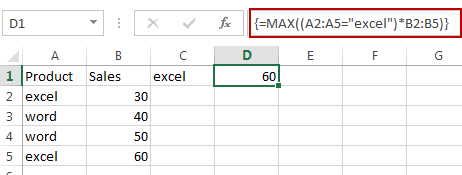You can also use another array formula based on the MAX function and the IF function to achieve the same result. Like this:

`=MAX(IF(A2:A5="excel",B2:B5))`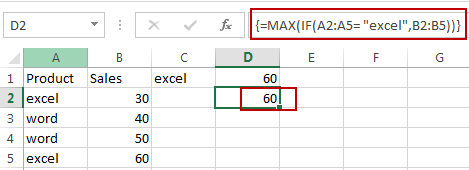## Find Max Value based on Multiple Criteria

Assuming that you want to find the maximum value of product “excel” from the month of Jan in range A1:D5, you can use the following array formula based on the MAX function and the IF function to find the max value based on multiple criteria. Like this:

`=MAX(IF(A2:A5="Jan", IF(B2:B5="excel",D2:D5),))`

Then you need to type this formula into a blank cell, and press Ctrl + Shift + Enter keys to change it as array formula. the Maximum value of product “excel” in the month of Jan would be returned.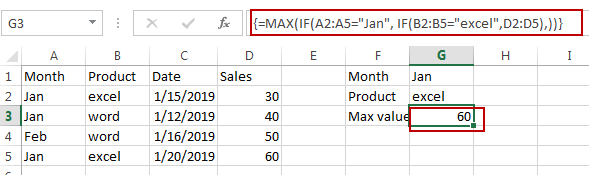## Find Min Value based on Single Criteria

If you want to find the minimum value of the product “excel” from the range A1:B5, you can use the following formula based on the MIN function:

`=MIN(IF(A2:A5="excel",B2:B5))`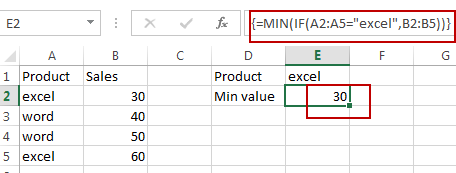## Find Min Value based on Multiple Criteria

If you want to find the minimum value of the product “excel” from the month of Jan in range A1:D5, you can use the following array formula based on the MIN function and the IF function to find the min value based on the multiple criteria. Like this:

`=MIN(IF(A2:A5="Jan",IF(B2:B5="excel",D2:D5)))`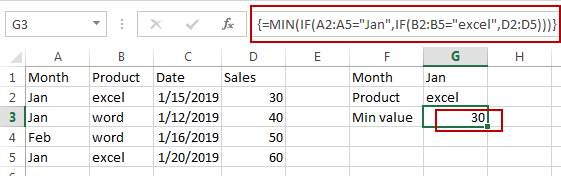### Related Functions

• Excel MIN function
The Excel MIN function returns the smallest numeric value from the numbers that you provided. Or returns the smallest value in the array.The MIN function is a build-in function in Microsoft Excel and it is categorized as a Statistical Function.The syntax of the MIN function is as below:= MIN(num1,[num2,…numn])….
• Excel MAX function
The Excel MAX function returns the largest numeric value from the numbers that you provided. Or returns the largest value in the array.= MAX(num1,[num2,…numn])…
• Excel IF function
The Excel IF function perform a logical test to return one value if the condition is TRUE and return another value if the condition is FALSE. The IF function is a build-in function in Microsoft Excel and it is categorized as a Logical Function.The syntax of the IF function is as below:= IF (condition, [true_value], [false_value])….